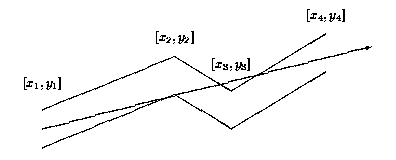# [POJ 1039] Pipe【计算几何】

• 2017-12-21
• 0
• 0

## Problem:

 Time Limit: 1000MS Memory Limit: 10000K

Description

The GX Light Pipeline Company started to prepare bent pipes for the new transgalactic light pipeline. During the design phase of the new pipe shape the company ran into the problem of determining how far the light can reach inside each component of the pipe. Note that the material which the pipe is made from is not transparent and not light reflecting.Each pipe component consists of many straight pipes connected tightly together. For the programming purposes, the company developed the description of each component as a sequence of points [x1; y1], [x2; y2], . . ., [xn; yn], where x1 < x2 < . . . xn . These are the upper points of the pipe contour. The bottom points of the pipe contour consist of points with y-coordinate decreased by 1. To each upper point [xi; yi] there is a corresponding bottom point [xi; (yi)-1] (see picture above). The company wants to find, for each pipe component, the point with maximal x-coordinate that the light will reach. The light is emitted by a segment source with endpoints [x1; (y1)-1] and [x1; y1] (endpoints are emitting light too). Assume that the light is not bent at the pipe bent points and the bent points do not stop the light beam.

Input

The input file contains several blocks each describing one pipe component. Each block starts with the number of bent points 2 <= n <= 20 on separate line. Each of the next n lines contains a pair of real values xi, yi separated by space. The last block is denoted with n = 0.

Output

The output file contains lines corresponding to blocks in input file. To each block in the input file there is one line in the output file. Each such line contains either a real value, written with precision of two decimal places, or the message Through all the pipe.. The real value is the desired maximal x-coordinate of the point where the light can reach from the source for corresponding pipe component. If this value equals to xn, then the message Through all the pipe. will appear in the output file.

Sample Input

```4
0 1
2 2
4 1
6 4
6
0 1
2 -0.6
5 -4.45
7 -5.57
12 -10.8
17 -16.55
0
```

Sample Output

```4.67
Through all the pipe.```

Source

Central Europe 1995

## Solution:

1. 采用 y = kx + b 表示直线能有效地降低编程复杂度，其中当光线垂直于x轴时可以直接退出
2. 特别注意浮点数的比较，不能直接用等于(==)、大于(>)和小于(<)，而是使用三态函数dsgn(x)，防止浮点误差导致结果出错。

## Code: O(n3) [184K, 47MS]

```#include<cstdio>
#include<cstdlib>
#include<cstring>
#include<cmath>
#include<iostream>
#include<algorithm>
#define eps 1e-8
#define dmax 1e10
using namespace std;

inline int dsgn(double x){
if(fabs(x) <= eps) return 0;
return (x < 0) ? -1 : 1;
}

#define Vector Point
struct Point{
double x, y;

Point() {}
Point(const double x, const double y): x(x), y(y) {}

inline Vector operator - (const Point p2) const {return Vector(x - p2.x, y - p2.y);}
};

struct Line2{
double k, b;

inline bool genLine(const Point &u, const Point &v){
double deltaX = v.x - u.x;
if(dsgn(deltaX) == 0) return 1;  // The slope doesn't exist
k = (v.y - u.y) / deltaX, b = u.y - k * u.x;
return 0;
}

inline double calcY(double x) {return k * x + b;}
};

int n;
Point up, dwn;
Line2 lin, curWall;
double ans;

inline double Cross(const Vector vec1, const Vector vec2) {return vec1.x * vec2.y - vec1.y * vec2.x;}

inline void tryLine(const Point &u, const Point &v){
if(ans > dmax - 1) return;  // No need to calculate more
if(lin.genLine(u, v)) return;
double curY = lin.calcY(up.x), curans = dmax;
if(dsgn(curY - up.y) == 1 || dsgn(curY - dwn.y) == -1) return;
// The light doesn't go through the entrance
// Beware of the double comparations, use the function "dsgn(x)" !!!!!!
for(register int i = 2; i <= n; i++){
curY = lin.calcY(up[i].x);
if(dsgn(curY - up[i].y) == 1){
curWall.genLine(up[i - 1], up[i]);
curans = (curWall.b - lin.b) / (lin.k - curWall.k);
break;
}  // The light is blocked by the upper pipe wall
if(dsgn(curY - dwn[i].y) == -1){
curWall.genLine(dwn[i - 1], dwn[i]);
curans = (curWall.b - lin.b) / (lin.k - curWall.k);
break;
}
}
ans = max(ans, curans);
}

int main(){
while(scanf("%d", &n) && n){
for(register int i = 1; i <= n; i++){
scanf("%lf%lf", &up[i].x, &up[i].y);
dwn[i].x = up[i].x, dwn[i].y = up[i].y - 1;
}
ans = -dmax;
for(register int i = 1; i <= n; i++)
for(register int j = i + 1; j <= n; j++){
tryLine(up[i], up[j]), tryLine(up[i], dwn[j]);
tryLine(dwn[i], up[j]), tryLine(dwn[i], dwn[j]);
}
if(ans > dmax - 1) puts("Through all the pipe.");
else printf("%.2f\n", ans);
}
return 0;
}
```

#### 评论

49750

https://github.com/Darkleafin

OPEN AT 2017.12.10

Please refresh the page if the code cannot be displayed normally.

https://visualgo.net/en

- Theme by Qzhai